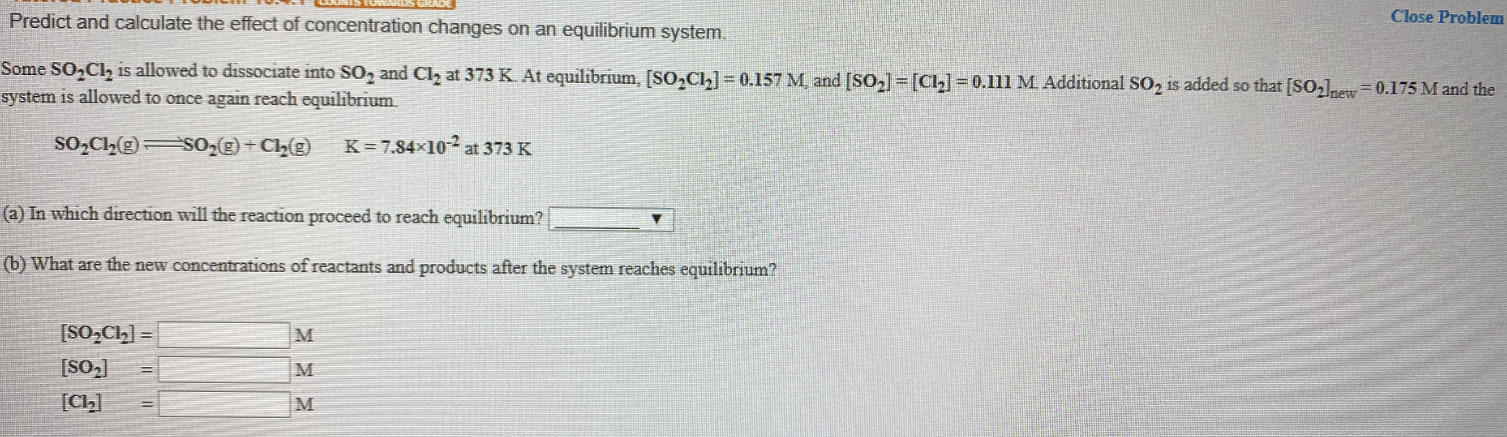# Predict and calculate the effect of concentration changes on an equilibrium system. Some SO2Cl is allowed to dissociate into SO2 and Cl2 at 373 K. At equilibrium, [SO2Cl2] = 0.157 M, and [SO2] = [Cl2] = 0.111 M. Additional SO2 is added so that [SO2]new = 0.175 M and the system is allowed to once again reach equilibrium. SO2Cl2(g) ⇌ SO2(g) + Cl2(g) K= 7.84x10^-2 at 373 K (a) In which direction will the reaction proceed to reach equilibrium? (b) What are the new concentrations of reactants and products after the system reaches equilibrium?